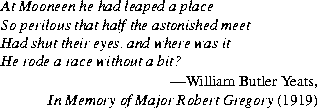Contents | Prev | Next | Index

# 21.2 The Class `java.util.BitSet`

A `BitSet` object is a set of bits that grows as needed. The bits of a `BitSet` are indexed by nonnegative integers. Each bit can be individually examined, set, or cleared. One `BitSet` may be used to modify the contents of another `BitSet` through logical AND, logical inclusive OR, and logical exclusive OR operations.

```public final class `BitSet` implements Cloneable {
public `BitSet`();
public `BitSet`(int nbits);
public String `toString`();
public boolean `equals`(Object obj)
public int `hashCode`();
public Object `clone`();
public boolean `get`(int bitIndex);
public void `set`(int bitIndex);
public void `clear`(int bitIndex);
public void `and`(BitSet set);
public void `or`(BitSet set);
public void `xor`(BitSet set);
public int `size`();
}
```

21.2.1 `public BitSet()`

This constructor initializes a newly created `BitSet` so that all bits are clear.

21.2.2 `public BitSet(int nbits)`

This constructor initializes a newly created `BitSet` so that all bits are clear. Enough space is reserved to explicitly represent bits with indices in the range `0` through `nbits-1`.

21.2.3 `public String toString()`

For every index for which this `BitSet` contains a bit in the set state, the decimal representation of that index is included in the result. Such indices are listed in order from lowest to highest, separated by "`, `" (a comma and a space) and surrounded by braces, resulting in the usual mathematical notation for a set of integers.

Overrides the `toString` method of `Object` (§20.1.2).

Example:

```BitSet drPepper = new BitSet();
```
Now `drPepper.toString()` returns `"{}"`.

```drPepper.set(2);
```
Now `drPepper.toString()` returns `"{2}"`.

```
drPepper.set(4);
drPepper.set(10);
```
Now `drPepper.toString()` returns `"{2, 4, 10}"`.

21.2.4 `public boolean equals(Object obj)`

The result is `true` if and only if the argument is not `null` and is a `BitSet` object such that, for every nonnegative `int` index `k`:

```((BitSet)obj).get(k) == this.get(k)
```
Overrides the `equals` method of `Object` (§20.1.3).

21.2.5 `public int hashCode()`

The hash code depends only on which bits have been set within this `BitSet`. The algorithm used to compute it may be described as follows.

Suppose the bits in the `BitSet` were to be stored in an array of `long` integers called, say, `bits`, in such a manner that bit `k` is set in the `BitSet` (for nonnegative values of `k`) if and only if the expression:

```
((k>>6) < bits.length) &&
((bits[k>>6] & (1L << (bit & 0x3F))) != 0)
```
is true. Then the following definition of the `hashCode` method would be a correct implementation of the actual algorithm:

```
public synchronized int hashCode() {
long h = 1234;
for (int i = bits.length; --i >= 0; ) {
h ^= bits[i] * (i + 1);
}
return (int)((h >> 32) ^ h);
}
```
Note that the hash code value changes if the set of bits is altered.

Overrides the `hashCode` method of `Object` (§20.1.4).

21.2.6 `public Object clone()`

Cloning this `BitSet` produces a new `BitSet` that is equal to it.

Overrides the `clone` method of `Object` (§20.1.5).

21.2.7 `public boolean get(int bitIndex)`

The result is `true` if the bit with index `bitIndex` is currently set in this `BitSet`; otherwise, the result is `false`.

If `bitIndex` is negative, an `IndexOutOfBoundsException` is thrown.

21.2.8 `public void set(int bitIndex)`

The bit with index `bitIndex` in this `BitSet` is changed to the "set" (`true`) state.

If `bitIndex` is negative, an `IndexOutOfBoundsException` is thrown.

If `bitIndex` is not smaller than the value that would be returned by the `size` method (§21.2.13), then the size of this `BitSet` is increased to be larger than `bitIndex`.

21.2.9 `public void clear(int bitIndex)`

The bit with index `bitIndex` in this `BitSet` is changed to the "clear" (`false`) state.

If `bitIndex` is negative, an `IndexOutOfBoundsException` is thrown.

If `bitIndex` is not smaller than the value that would be returned by the `size` method (§21.2.13), then the size of this `BitSet` is increased to be larger than `bitIndex`.

21.2.10 `public void and(BitSet set)`

This `BitSet` may be modified by clearing some of its bits. For every nonnegative `int` index `k`, bit `k` of this `BitSet` is cleared if bit `k` of `set` is clear.

21.2.11 `public void or(BitSet set)`

This `BitSet` may be modified by setting some of its bits. For every nonnegative `int` index `k`, bit `k` of this `BitSet` is set if bit `k` of `set` is set.

21.2.12 `public void xor(BitSet set)`

This `BitSet` may be modified by inverting some of its bits. For every nonnegative `int` index `k`, bit `k` of this `BitSet` is inverted if bit `k` of `set` is set.

21.2.13 `public int size()`

This method returns the number of bits of space actually in use by this `BitSet` to represent bit values.Java Language Specification (HTML generated by Suzette Pelouch on February 24, 1998)